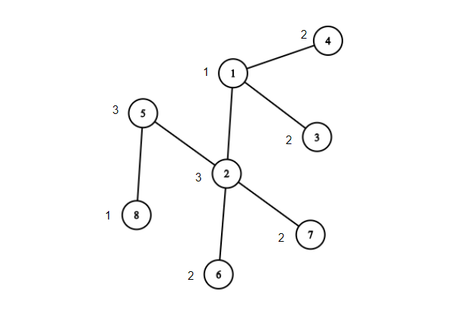# Race in DomKiprodip_bsmrstutest-2 contest
Limits 1s, 512 MB

There is a kingdom called DomKi. DomKi has N city and N-1 two-way road. Each city is numbered with 1, 2, 3, … N. The cities of DomKi are connected to each other in such a way that it forms a [Tree](https://en.wikipedia.org/wiki/Tree_(graph_theory). Each city in DomKi is given a rank, r which is an integer.

Recently the Tournament Planning Committee(TPC) of the kingdom decided to arrange a car race in the Kingdom. In this tournament, racers from all over the world are invited to take part. So the TPC is a bit tense to arrange a successful tournament because finishing a tournament successfully is not an easy task.
The most important issue is the information about the tracks where the race will be held. They are so eager to know the details of the tracks before the tournament.
Note, that a track is consist of one or more roads. For better understanding, you can consider the track as the shortest route between two cities in DomKi.
Let me introduce one more thing about the track.
Suppose for a track the TPC has fixed two cities a and b where a is the starting city of the track and b is the city where the track end and called this track T(a,b). For a track T(a,b), if we store all the ranks r lying on the track T(ab) and write them in a paper sequentially, then it will be an array and TPC called this array Rank Array of the track Tab, (RA(a,b)).

They, basically want to know three important facts about DomKi’s tracks.
They listed the information, what they want to know, in a structured way like below.

• 1 a b - Is it possible to make RA(a,b) a palindrome array by rearranging(not necessarily) the elements of the array RA(a,b)?
• 2 a b - Is the array RA(a,b) a palindrome array ?
• 3 a b c d - Are the array RA(a,b) and RA(c,d)) identical ?

To understand better, please see the explanation of sample case.
Meanwhile, the TPC have managed to know that you are a great programmer of your country, and they are planning to hire you.
Now the question is, can you help them to answer the query regarding the tracks?

## Input

The input will start with an integer T (T ≤ 12), denoting the number of test cases.
For each test case,
In the first line, you will be given an integer N (1 ≤ N ≤ 2×105), the number of cities in DomKi. The next line will contain N integers r1, r2, r3 ... rN, the ranks of the cities(1 ≤ ri ≤ 60) where for every i(1 ≤ i ≤ N), ri is the rank of the ith city.
Each of the next N - 1 lines will contain two integers u v, which means there is a two-way road between city u and v.
The next line will contain an integer Q(1 ≤ Q ≤ 2×105), the number of questions the TPC will ask.
Each of the next Q lines will contain a question of type 1, 2 or 3.
It is guaranteed that there can be at most one road between any pair of cities and no road connects itself. Also, it is possible to go from each city to any other city by using some sequence of roads.
Sum of N over all test cases won't exceed 400000
Sum of Q over all test cases won't exceed 550000

## Output

For each test case, first print the line, "Case X:", where X is the case number and then for each query, print "YES" or "NO" (without quotes) depending on the question of the TPC, in a new line.
See the sample output for formatting.

## Sample

InputOutput
1
8
1 3 2 2 3 2 2 1
8 5
6 2
5 2
3 1
1 4
1 2
2 7
6
1 5 6
1 6 8
2 1 8
2 5 7
3 3 8 4 8
3 4 5 3 7

Case 1:
YES
NO
YES
NO
YES
NO


The structure of the kingdom, DomKi.For query 1, RA(5,6) = {3, 3, 2}. By rearranging the order of the values we can make it as, RA(5,6) = {3, 2, 3}, which is palindrome. So the answer is YES.
For query 2, RA(6,8) = {2, 3, 3, 1}. It's not possible to make it palindrome by changing the order of the elements. So the answer is NO.
For query 3, RA(1,8) = {1, 3, 3, 1} which is a palindrome. So the answer is YES.
For query 4, RA(5,7) = {3, 3, 2} which is not a palindrome. So the answer is NO.
For query 5, RA(3,8) = {2, 1, 3, 3, 1} and RA(4,8) = {2, 1, 3, 3, 1}. Here the size of RA(3,8) and size of RA(4,8) are equal and for every i (1 ≤ i ≤ size(RA(3,8))) RA(3,8)[i] == RA(4,8)[i]. So RA(3,8) is identical to RA(4,8).Note, We considered both array as 1-indexed.
For query 6, RA(4,5) = {2, 1, 3, 3} and RA(3,7) = {2, 1, 3, 2}. Here the size of RA(4,5) and size of RA(3,7) are equal but for i = 4, RA(3,7)[i] != RA(4,5)[i]. So RA(4,5) is not identical to RA(3,7).uDebug

### Submit